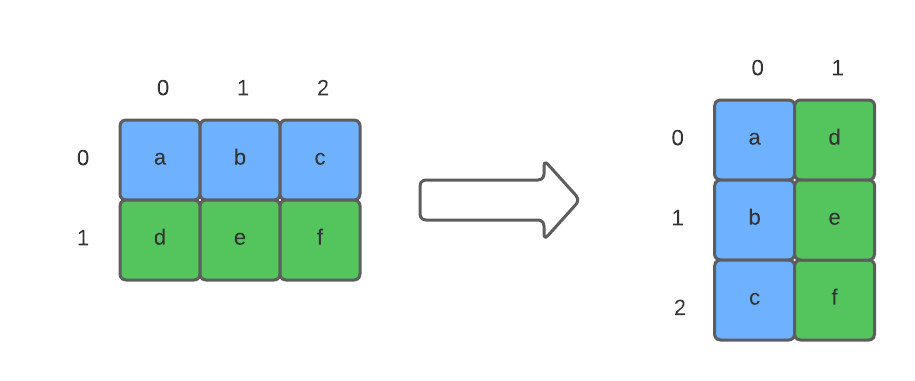Computer Science

# Grid coordinates

We can think of a grid as a two-dimensional structure that can be accessed using coordinates `(row, column)`:The cell that is colored blue has coordinates `(1, 2)`, meaning it is in row 1, column 2.

## Using coordinates to access grid cells

Let’s write a `print_grid()` function that uses coordinates to access each cell:

``````def print_grid(grid):
for row in range(len(grid)):
for column in range(len(grid[row])):
print(grid[row][column], end='\t')
print()``````
• `len(grid)` = number of rows in the grid
• `grid[row]` = a specific row of the grid, e.g. `grid` = row 1
• `len(grid[row])` = number of columns in a specific row of the grid
• `grid[row][column]` = item in the cell that is at location `(row, grid)`
• `end='\t'` = prints a tab character after the contents of each cell

If we call this function:

``````numbers = [
[1, 2, 3, 4],
[5, 6, 7, 8],
[9, 10, 11, 12]
]
print_grid(numbers)``````

then it will print:

``````1	2	3	4
5	6	7	8
9	10	11	12``````

## Traversing a grid by columns instead of rows

What happens if we change the order of the `for` loops in our `print_grid()` function?

``````def print_grid_by_columns(grid):
for column in range(len(grid)):
for row in range(len(grid)):
print(grid[row][column], end='\t')
print()``````

If we run this with the grid above, we get:

``````1	5	9
2	6	10
3	7	11
4	8	12``````

The order of the loops controls the direction the grid is traversed.

## Averaging the values of each row

Let’s write a function that computes the average of each row of a grid. It should return the averages in a list.

``````def row_average(grid):
result = []
for row in grid:
result.append(sum(row) / len(row))
return result``````

We are using the built-in function `sum()`, which can sum all the elements of a list.

If we run this function:

``````numbers = [
[1, 2, 3, 4],
[5, 6, 7, 8],
[9, 10, 11, 12]
]
print(row_average(numbers))``````

we get:

``[2.5, 6.5, 10.5]``

## Computing the maximum length of a string in a column

Working on columns is a little bit more challenging. Suppose we have a grid of strings:

``````fruits = [
['apple', 'pear', 'guava'],
['orange', 'banana', 'peach'],
['melon', 'mango', 'kiwi']
]``````

Let’s write a function that computes the maximum length of the strings in each column, returning those maximums in a list.

``````def column_max_string_length(grid):
"""Returns the maximum length of the strings in each column. Assumes the grid is regular."""
maximums = []
for column in range(len(grid)):
max_length = None
for row in range(len(grid)):
item_length = len(grid[row][column])
if max_length is None or item_length > max_length:
max_length = item_length
maximums.append(max_length)
return maximums``````

We have to loop through column to compute its maximum. To get the number of columns we use `len(grid)`. As we look at a given column, we can loop through all the rows and check the length of the item in that particular column.

If we run this code:

``````fruits = [
['apple', 'pear', 'guava'],
['orange', 'banana', 'peach'],
['melon', 'mango', 'kiwi']
]
print(column_max_string_length(fruits))``````

the output is:

``[6, 6, 5]``

## Transposing a grid

Transposing a grid means flipping it diagonally:Notice how `row 0` becomes `column 0`, and `row 1` becomes `column 1`.

One way to do this is to copy each row into a column, and then append those columns into a new grid. Here is a solution that does that:

``````def transpose(grid):
"""Returns a tranposed copy of the grid."""
new_grid = []
# loop through all of the columns
for column in range(len(grid)):
# create a column
new_column = []
# loop through a row and append its values to a column
for row in range(len(grid)):
new_column.append(grid[row][column])
# append the column to the grid
new_grid.append(new_column)
return new_grid``````

If we run this:

``````my_grid = [
[1, 2],
[3, 4],
[5, 6],
[7, 8]
]
print_grid(my_grid)
print_grid(transpose(my_grid))``````

the output is:

``````1	3	5	7
2	4	6	8``````

## An alternative transpose solution

A different way to think about this is to create a new grid that has the right size we need, filled with `None`. This new grid needs its `rows` to be equal to the number of `columns` in the original grid. Likewise, its `columns` should be equal to the number of `rows` in the original grid. We can then copy the values from each row into each column.

``````def make_empty_grid(rows, columns):
new_grid = []
for row in range(new_num_rows):
new_row = []
for column in range(new_num_columns):
new_row.append(None)
new_grid.append(new_row)
return new_grid

def transpose2(grid):
"""Returns a transposed copy of the grid."""

num_rows = len(grid)
num_columns = len(grid)

# make a new grid that is the right shape
new_grid = make_empty_grid(num_columns, num_rows)

# populate the new grid
for row in range(num_rows):
for column in range(num_columns):
new_grid[column][row] = grid[row][column]

return new_grid``````

• `sum()` - computes the sum of a list of numbers
• `max()` - computes the maximum of a list of numbers
• `min()` - computes the minimum of a list of numbers
``````numbers = [1, 2, 3, 4, 5]